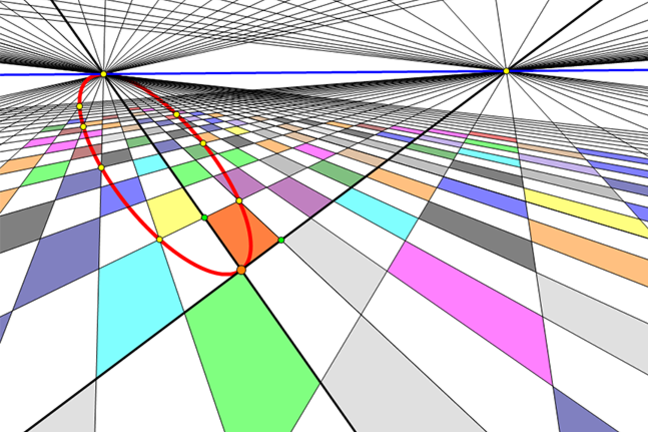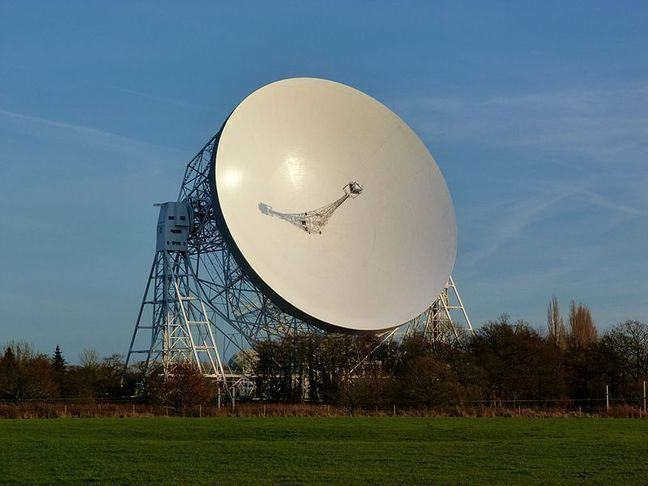## Want to keep learning?

This content is taken from the UNSW Sydney's online course, Maths for Humans: Linear and Quadratic Relations. Join the course to learn more.
3.13

## UNSW SydneyA parabola in perspective view

# Symmetry, foci and directrices for standard parabolas

The parabola plays a key role in mathematics, as it is the shape of a quadratic function. The simplest examples are the standard parabolas with Cartesian equations $$\normalsize{y=ax^2}$$.

In this step we

• explain the role of the axis and reflective property of the parabola that is important in many applications

• examine the focus/directrix definition of a parabola using Cartesian coordinates.

## The standard parabola and quadratic function

The most familiar parabola in Cartesian coordinates has equation $$\normalsize{y=x^2}$$, which corresponds to the function

$\Large{f(x)=x^2}.$

It passes through the origin $$\normalsize{[0,0]}$$, as well as the points $$\normalsize{[1,1]}$$, $$\normalsize{[-1,1]}$$, $$\normalsize{[2,4]}$$, $$\normalsize{[-2,4]}$$ and so on.We observe that this parabola is symmetric: the $$\normalsize{y}$$-axis is a line of symmetry, in other words the reflection $$\normalsize{[x,y]}$$ goes to $$\normalsize{[-x,y]}$$ takes one point on the parabola to another. This line is the axis of the parabola. The point where the axis meets the parabola is the vertex, and this is clearly the origin $$\normalsize{[0,0]}$$.

Let’s also observe that for small values of $$\normalsize{x}$$ close to $$\normalsize{0}$$, the $$\normalsize{y}$$ values of a point $$\normalsize{[x,y]}$$ on the parabola are even much smaller, so the parabola hugs the $$\normalsize{x}$$-axis. We say that the $$\normalsize{x}$$-axis is the tangent line to the parabola at the origin $$\normalsize{[0,0]}$$.

Q1 (E): If $$\normalsize{x=0.001}$$ then what is the value of $$\normalsize{y=f(x)=x^2}$$? How about if $$\normalsize{x=-0.00002}$$?

As $$\normalsize{x}$$ increases beyond $$\normalsize{1}$$, the quadratic nature of the curve means that $$\normalsize{y}$$ increases faster and faster. So for larger values of $$\normalsize{x}$$, the $$\normalsize{y}$$-values are much bigger.

Q2 (E): If $$\normalsize{x=10000}$$ then what is $$\normalsize{y}$$? How about if $$\normalsize{x=2,000,000}$$?

## Zooming out for a better look

One way of getting a better look at the parabola is to zoom out — that is, to rescale. In the zoomed out graph below, you see that from farther out the parabola looks skinnier!If you examine the image at the top of this step, you will see the parabola in a perspective view that an artist might draw using a horizon.

Q3 (M): In that figure, can you locate the $$\normalsize{x}$$- and $$\normalsize{y}$$-axes? Can you still see the same points that the parabola passes through in this perspective image? The parabola appears to us as an ellipse. Would this have been a surprise to Apollonius?

## The axis and the reflective property of the parabola

The parabola has an important reflective property. If we had a mirror shaped like our parabola, then any light beam that travels parallel to the axis of the parabola will bounce off the parabola and travel through a particular point, called the focus $$\normalsize{F}$$.Similarly, any light beam traveling towards the mirror from the focus will reflect off the surface and travel along a path parallel to the axis. This is the reason that headlights of a car have a parabolic shape, as they produce a coherent beam of light focussed in one direction. It is also the reason that satellite dishes and some types of telescopes have a parabolic shape: they take parallel incoming beams and focus them to a point where they can be picked up by a receiver.© “Lovell telescope” by Delusion23/Wikimedia Commons CC BY SA

## Definition of a parabola in terms of focus and directrix

Apollonius knew that a parabola could also be defined as the locus (a somewhat old fashioned word meaning path, or trajectory) of a point $$\normalsize{X}$$ whose distance from a fixed point $$\normalsize{F}$$ equals its distance from a fixed line $$\normalsize{l}$$. Using the rational concept of quadrance, whose square root is the distance, it is algebraically simpler to state this condition in the form

$\Large{Q(X,F)=Q(X,l). \tag 1}$

The point $$\normalsize{F}$$ here is exactly the same focus of the parabola that figured in the reflective property above!! The line $$\normalsize{l}$$ is called the directrix. In fact the ellipse and hyperbola have a similar description, as we shall see in a while.In the figure we see the parabola

$\Large{y=\frac{1}{4} x^2}$

with its focus the point $$\normalsize{F=[0,1]}$$ and its directrix the line $$\normalsize{l: y=-1}$$. The coefficient $$\normalsize{1/4}$$ has the effect of rescaling the parabola in the $$\normalsize{y}$$ direction: making it a bit wider than $$\normalsize{y=x^2}$$. This is a nice parabola because its focus and directrix have such a simple form.

## A calculation

In this section, let’s verify that the quadrance condition (1) defining the parabola $$\normalsize{y=x^2/4}$$ really works. A general point on this parabola is $$\normalsize{X=[x,x^2/4]}$$. Then using Pythagoras’ theorem for quadrance between $$\normalsize X$$ and $$\normalsize F=[0,1]$$, we have

$\Large{Q(X,F)=(x-0)^2+(x^2/4-1)^2=x^4/16+x^2/2+1}$

where we have used the algebraic rule

$\Large{(a-b)^2=a^2-2ab+b^2}.$

On the other hand, since the difference in the $$\normalsize{y}$$ coordinates between the point $$\normalsize{[x,x^2/4]}$$ and the line $$\normalsize{y=-1}$$ is $$\normalsize{x^2/4+1}$$, we see that

$\Large{Q(X,l)=(x^2/4+1)^2=x^4/16+x^2/2+1}.$

It follows that yes, these two quadrances are always equal, no matter which point $$\normalsize{X}$$ we choose.

## The focus and directrix of $$\normalsize{y=x^2}$$

It turns out that the focus of $$\normalsize{y=x^2}$$ is

$\Large{F=[0,\frac{1}{4}]}$

and its directrix $$\normalsize{l}$$ is the line

$\large{y=-1/4}.$Q4 (C): See if you can verify this algebraically, following our computation above.

A1. If $$\normalsize{x=0.001}$$ then $$\normalsize{y=(0.001)^2=0.000001}$$. And if $$\normalsize{x=-0.00002}$$ then $$\normalsize{y=(-0.00002)^2=0.0000000004=4 \times 10^{-10}}$$.

A2 If $$\normalsize{x=10000}$$ then $$\normalsize{y=(10000)^2=100000000=10^8}$$. And if $$\normalsize{x=2,000,000}$$ then $$\normalsize{y=(2,000,000)^2=(2 \times 10^6)^2=4 \times 10^{12}}$$.

A3. The $$\normalsize{x}$$- and $$\normalsize{y}$$-axes are marked by darker black lines. Apollonius would not have been surprised. The cone of light from our eye to the parabola is indeed just a cone like he studied, and its intersection with the horizontal plane which we are calling the $$\normalsize{x-y}$$ plane is, in this case, an ellipse.

A4. We hope your computation succeeded. If not, have another look at our computation and follow it carefully.